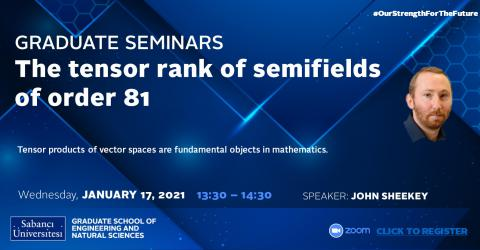## SEMINAR:The tensor rank of semifields of order 81

### SEMINAR:The tensor rank of semifields of order 81Speaker: John Sheekey

Title:The tensor rank of semifields of order 81

Date/Time: 17 March 2021 / 13:30 - 14:30

Passcode:-  algebra

Abstract: Tensor products of vector spaces are fundamental objects in mathematics. The tensor product of two vector spaces can be studied using matrices, and this case is well-understood; the rank can be calculated easily, and equivalence corresponds precisely with rank. However for higher order tensors, problems such as calculating the rank or determining equivalence becomes very difficult.The case of the tensor product of three isomorphic vector spaces corresponds to algebras in which multiplication is not assumed to be associative. In this case, the tensor rank gives an important measure of the complexity of the multiplication in the corresponding algebra. For the case of a finite semifield (i.e. a not-necessarily associative division algebras), lower bounds can be obtained using results from linear codes, while for field extensions upper bounds can be obtained via polynomial interpolation and algebraic geometry.

In this talk we will survey these problems and present new results where we determine the tensor rank of all finite semifields of order 81. In particular we show that some semifields of order 81 have lower tensor rank than the field of order 81, the first known example of such a phenomenon.

Bio: john Sheekey was awarded his PhD from University College Dublin in 2012, with thesis titled "On Rank Problems for Subspaces of Matrices over Finite Fields". He subsequently undertook Postdoctoral positions at Università di Padova, VUB (Brussels), and Ghent University, with the latter as an FWO fellow. In 2015 he returned to UCD as Assistant Professor, and since 2019 he has had the position of Assistant Professor - Ad Astra Fellow. John's research interests lie in linear and multilinear algebra over finite fields, in particular semifields, rank-metric codes, and tensors.